+

Author: Sophia Tutorial
##### Description:

Determine the graph of a quadratic inequality.

(more)

Sophia’s self-paced online courses are a great way to save time and money as you earn credits eligible for transfer to many different colleges and universities.*

No credit card required

37 Sophia partners guarantee credit transfer.

299 Institutions have accepted or given pre-approval for credit transfer.

* The American Council on Education's College Credit Recommendation Service (ACE Credit®) has evaluated and recommended college credit for 32 of Sophia’s online courses. Many different colleges and universities consider ACE CREDIT recommendations in determining the applicability to their course and degree programs.

Tutorial
what's covered
1. Graphing a Parabola
2. Graphing an Inequality

# 1. Graphing a Parabola

More than likely, you'll be given a quadratic equation or inequality in standard form: ax2 + bx + c. To graph this, it is helpful to find the vertex to the parabola, and then plot some points on one side of the vertex. Since the vertex lies on the axis of symmetry, we can simply reflect the already plotted points across this line, to create a more complete sketch of the parabola.

To find the vertex and/or axis of symmetry when working in standard form, we use the formula: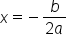, where a and b come from the coefficients of the x-squared and x-terms in standard form.

The previous formula is the equation to the vertical line which is the axis of symmetry (for vertical parabolas). This also represents the x–coordinate of the vertex. To find the y–coordinate, simply use the calculated value of x in the original equation, and solve for y.

# 2. Graphing an Inequality

Graphing inequalities is similar to graphing equations, but there are some important differences. First, there is a difference between using a solid line and a dashed line to draw the curve. If the inequality symbol is strict ( < or >) we always use a dashed line to show that we are not including exact values on the boundary line. If the inequality symbol is non-strict ( ≤ or ≥ ) we always use a solid line to show that we are including exact values on the boundary line.

Not only do we need to be careful about what type of line to use when drawing the graph, we also need to highlight a solution region. The highlighted solution region represents the set of all (x, y) coordinates that satisfy the inequality. To decide which inequality region to highlight, we use a test point. This test point can be anywhere on the coordinate plane, so long as it isn't on the boundary line (because that would not help us at all). We take the x– and y– values from this test point and plug it into our inequality. If the statement is true, the half-plane that the test point lies within is the solution region, and gets highlighted. If not, the other half-plane gets highlighted.

# 3. Graphing a Quadratic Inequality

Let's apply what we know about graphing parabolas and graphing inequalities to graph a quadratic inequality. Let's graph the following inequality: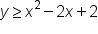The first thing we need to do is graph the boundary line, y = x2 – 2x + 2. We will use a solid line to graph the parabola, because our inequality symbol is non-strict. To graph the boundary line, we need to find the vertex, plot a couple of points, and then reflect them across the axis of symmetry: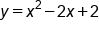Equation to the boundary line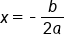Equation to the axis of symmetry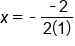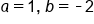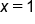Location of axis of symmetry

We use x = 1 to find the y–coordinate of the vertex:Equation to the boundary line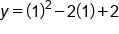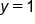y-coordinate of vertex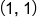Vertex

Next, we plot a few points on one side of the vertex, and reflect them across the axis of symmetry to sketch the boundary line:

 xy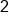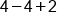2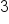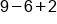5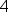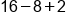10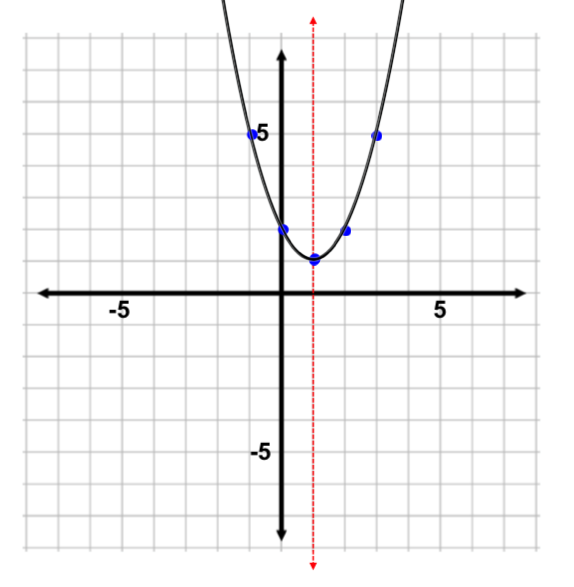The final step is to choose any test point (not on the boundary line) to plug x– and y–values into the inequality. Remember, a true statement means the point lies within the solution region (and that half-plane should be shaded). Otherwise, the other half-plane gets shaded.

hint
Use the test point (0, 0) if you can. This is because it is easy to multiply by zero and add zero to quantities. It is always recommended to make calculations simpler!Our inequality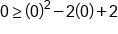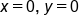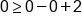Evaluate terms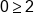False statement

Since the point (0, 0) yields a false statement, we highlight the other region of the coordinate plane as our solution region (we do not highlight the region that includes the origin (0,0) in this case):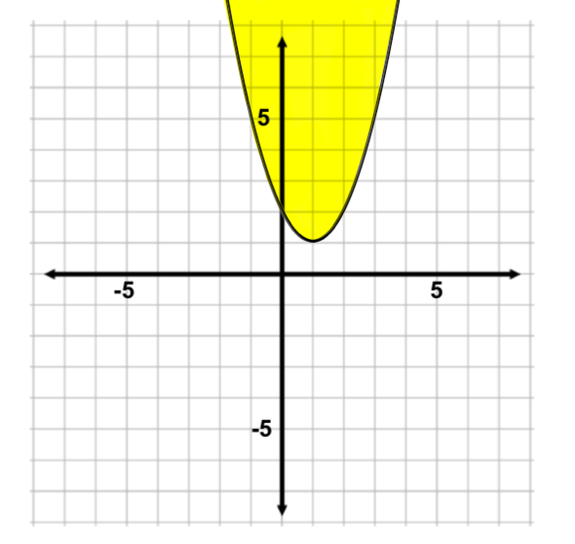summary
To graph a quadratic inequality, we graph the parabola as we do with equations, but use either a dotted or a solid line, depending on the inequality. When graphing an inequality, the inequality symbols less than and greater than indicate using dotted or dashed lines, and inequality symbols less than or equal to or greater than or equal to indicate using solid lines. When graphing a quadratic inequality, the solution to the inequality can be represented by shading a portion of the plane. If a test point yields a true inequality statement, then the region the point lies in is shaded. If a test point yields a false inequality statement, then the other portion of the plane is shaded as the solution region.
Rating